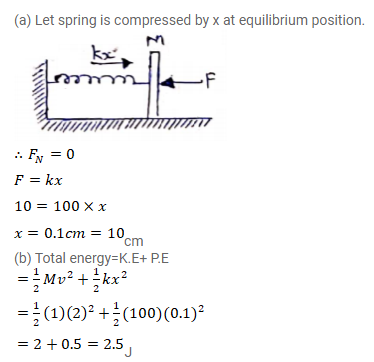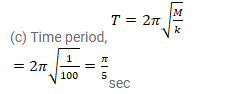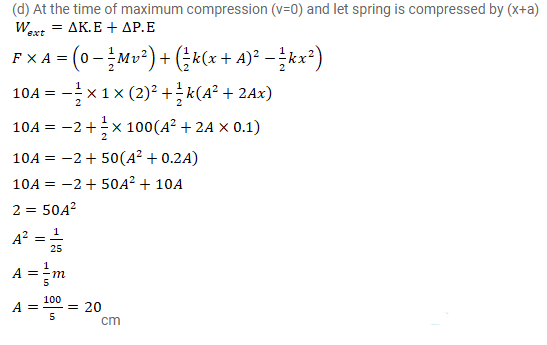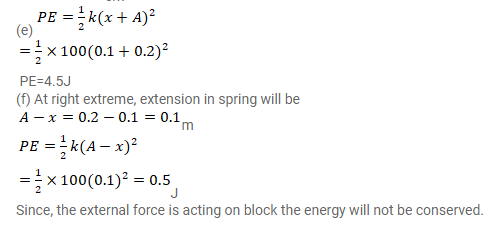# In figure k=100N/m, M=1kg and F=10N.

Question:

In figure $\mathrm{k}=100 \mathrm{~N} / \mathrm{m}, \mathrm{M}=1 \mathrm{~kg}$ and $\mathrm{F}=10 \mathrm{~N}$.

(a) Find the compression of the spring in the equilibrium position.

(b) A sharp blow by some external agent imparts a speed of $2 \mathrm{~m} / \mathrm{s}$ to the block towards left. Find the sum of the potential energy of the spring and the kinetic energy of the block at this instant.

(c) Find the time period of the resulting simple harmonic motion.

(d) Find the amplitude.

(e) Write the potential energy of the spring when the block is at left extreme.

(f) Write the potential energy of the spring when the block is at the right extreme.

The answers of (b), (e) and (f) are different. Explain why this does not violate the principle of conservation of energy.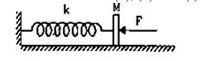Solution: